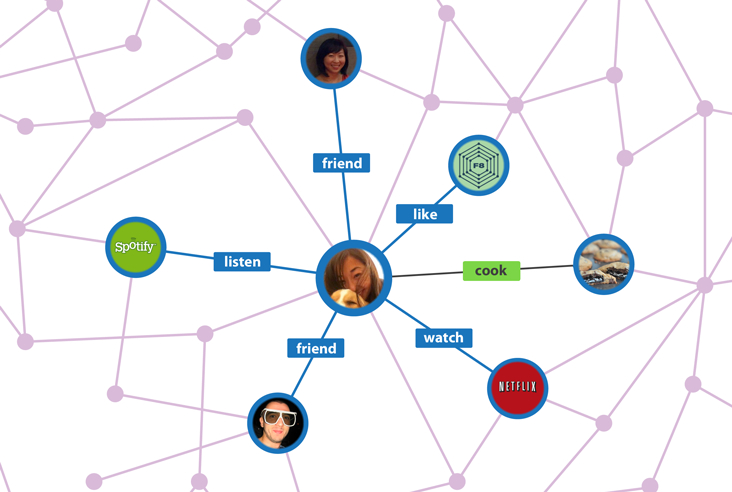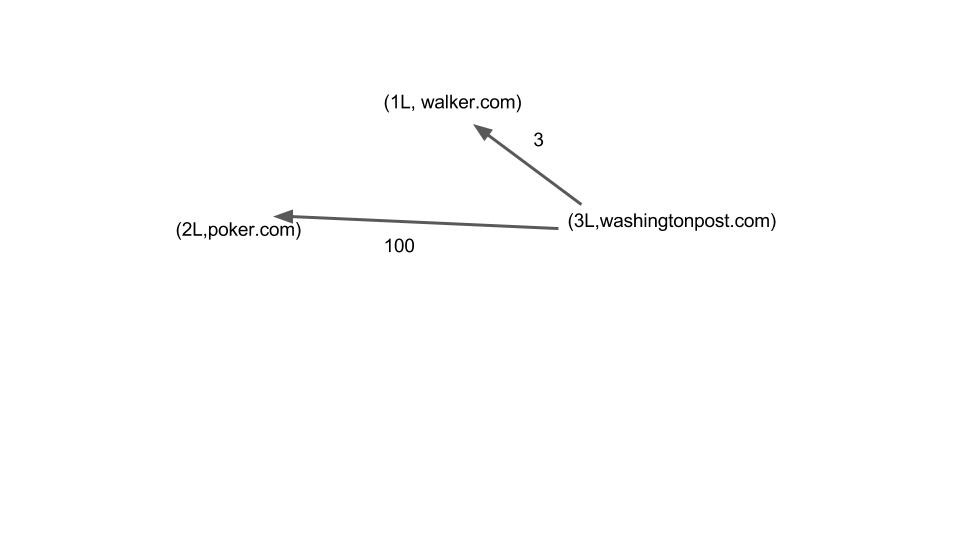The Spark GraphX API is a graph processing API built on top Apache Spark. Since it is built on top of Spark, it is built to process graphs across a distributed architecture.

“Graphs” here mean graphs from graph theory as opposed to graphs like those used to show profit growth. Graphs in the mathematical sense are sets of vertices and edges. The most common example use to explain that is modelling the relationship between people in a social network. In fact, Facebook’s API for accessing that is called the Facebook Graph API. Another common example in Google’s Search engine algorithm, called the PageRank Index.

For example, here is a small depiction of that taken from an article by Ted Nguyen on the Facebook API.Here the purple lines are edges and the people are vertices. An edge can have attributes, like “like.” Vertices can have attributes as well, like someone’s name.

Using the graph, in the Facebook example, it is possible to know who is friends with who and what interests do they have in common, since they have liked certain pages. That is important for many things, such as targeted advertising.

To translate this to Apache Spark and Scala look at this graph that shows how many links one website has to another. In this example there are just 3 websites and 2 link counters.Here we have three Vertices, which we write as Scala objects like this:

import org.apache.spark.graphx._

var webSites = Array((1L, “walker.com”), (2L, “poker.com”), (3L, “washingtonpost.com”))

There can be more than one attribute for each vertex. Here there is just one: the domain name. And the index is a long, which is a 64 bit number, because there are many websites.

var edges = Array(Edge(3L,1L,3),Edge(3L,2L,100))

Here each Edge has the from and the to vertex number. The attribute is the number of links between each of these sites. This is taking a shortcut as computing that is one thing the PageRank algorithm does.

To make a graph object we first have to turn these objects into RDDs:

var edgesRDD = sc.parallelize(edges)
var webSitesRDD = sc.parallelize(webSites)

var webSitesRDD = sc.parallelize(webSites)

Now we can print each vertex like this:

rank.vertices.collect.foreach { case (id, website) => println(s”\$id \$website”)}

The vertex and the edges also make a GraphX Triplet. The triplet extends by Edge class by adding srcAttr (source attribute) and dstAttr (destination attribute). So we can print those like this:

rank.triplets.collect.foreach { e => println(s”\${e.srcAttr} is linked to \${e.dstAttr}”)}